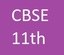Trending ▼   ResFinder# CBSE Class 11 Pre Board 2019 : Computer Science (St Xavier's Sr. Sec. School, Delhi)

3 pages, 35 questions, 0 questions with responses, 0 total responses,00Cbse 11 +Fave Message ProfileTimelineUploads
 Home > cbse11 >   F Also featured on: School PageFormatting page ...

Annual Examination in COMPUTER SCIENCE Std. 11 28-2-2019 Time : 3 hrs. Max. Marks : 70 1. Write any two differences between each of the following  a) char array and array of strings b) Normal array and unsized arrays c) Arrays and structures d) strcmp( ) and strcpy( ) f) cout and puts( ) 2. a) Write two limitations of 2D unisized array.  b) Write declarations for the following: I. Array to store all houses names (eg. Tagore, Gandhi etc..) II. Array to store first five elements of Fibonacci series. c) Write headers files for built in function given below: i) cos( ) ii) toupper( ) iii) strcpy( ) iv) exit(0) v) randomize( ) vi) setprescision( ) d) How does c++ view string as? Which character marks end of the string?  e) Write one drawback of random( ) function? How you will remove this drawback?  f) Find total no of bytes required to store each of the following array.  i) char S[3+5]; ii) float f[30-5]; g) Write maximum number of elements 1D array and 2D array can hold.  h) What will be the value of the components of given arrays.  i) float sal={7899.00}; ii) char S ={ D }; iii) int D[ ]={88.5}; i) What is the size of the array: int data[] = { 12, 34, 9, 0, -62, 88 };  j) How many rows are there in the given array: float S[ ] ={ 2,3,5,6,78.5};  3 Underline and correct the errors in the given program segements. a) void main( ) { clrscr( ); float a[10.0]; char s[ ]= Hi ; int a{c}; for(i=0; i=5; i++) cin>>a; get(s); } b) void main() { struct student( ) { char name; int age=17; int roll=4; } Student stud; Strcpy(stud.name, Haris ); age=17; cout<<name; cout << age.stud; } 4. Write the output of the following. a) void main( ) {char x[ ] = InFOrMAtIOn ; int i=0; while( x[i] != \0 ) {if (i%2 = = 1) x[i] = tolower(x[i]); else x[i]= * ; i++; } cout<<x; } Std. 11 -2-    COMPUTER SCIENCEFormatting page ...Formatting page ...Tags : cbse class xi, cbse 11, cbse 11th standard, cbse mock papers, cbse sample papers, cbse books, portal for cbse india, cbse question bank, central board of secondary education, past cbse question papers with answers, prelims preliminary exams, pre board exam papers, cbse model test papers, solved board question papers of cbse last year, previous years solved question papers, free online cbse solved question paper, cbse syllabus, india cbse board sample questions papers, last 10 years cbse final exam papers, cbse question papers, cbse guess sample questions papers, cbse important questions, specimen / mock papers.